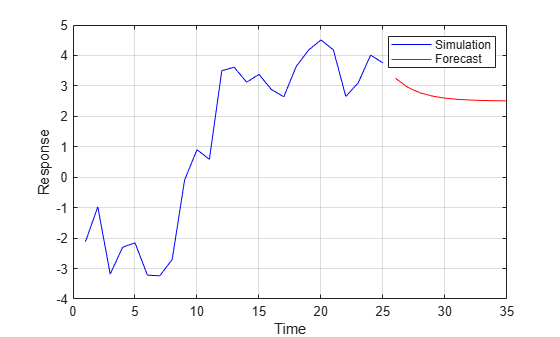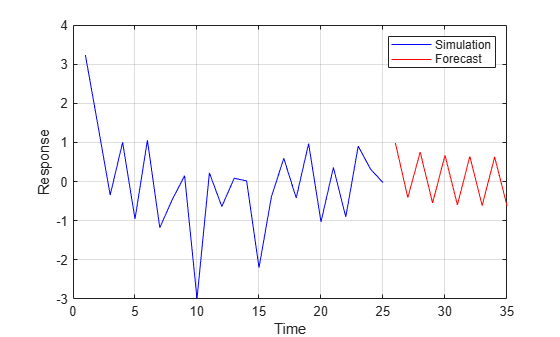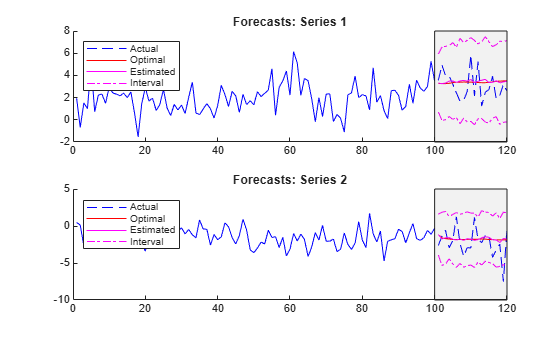# Forecast Threshold-Switching Dynamic Regression Models

This example shows how to forecast paths of threshold-switching models by using the `forecast` function.

### Generate Optimal One-Step-Ahead Forecasts

When you pass a fully specified model to `forecast` and return only the first output argument, `forecast` iteratively generates optimal one-step-ahead point forecasts. To illustrate this behavior, consider the threshold-switching model with the following characteristics:

• The state switches abruptly when the threshold variable ${\mathit{y}}_{\mathit{t}-1}$ changes sign.

• The state 1 (negative) submodel is ${\mathit{y}}_{\mathit{t}}=-1+0.6{\mathit{y}}_{\mathit{t}-1}+{\epsilon }_{\mathit{t}}$.

• The state 2 (nonnegative) submodel is ${\mathit{y}}_{\mathit{t}}=1+0.6{\mathit{y}}_{\mathit{t}-1}+{\epsilon }_{\mathit{t}}$.

• The model-wide innovation process ${\epsilon }_{\mathit{t}}\sim Ν\left(0,0.5\right)$.

Create the self-exciting threshold autoregressive (SETAR) model.

```tt0 = threshold(0); mdl1 = arima(Constant=-1,AR=0.6); mdl2 = arima(Constant=1,AR=0.6); Mdl12 = tsVAR(tt0,[mdl1 mdl2],Covariance=0.5);```

`Mdl12` is a fully specified `tsVAR` object representing the threshold-switching model. The model is agnostic of the threshold variable at this point, it is aware only of the characteristics of the threshold transition.

Simulate a path of 25 responses from the SETAR model.

```rng(0) % For reproducibility numObs = 25; ys = simulate(Mdl12,numObs);```

`ys` is a 25-by-1 vector representing a simulated path from `Mdl12`.

Generate iterative point forecasts of the conditional mean of the SETAR model into a 10-period forecast horizon by calling `forecast` and returning only the forecasted conditional means (the first output argument). Supply the simulated path as a presample for the forecast (the forecast period starts at time $\mathit{t}=26$).

```fh = 10; yf = forecast(Mdl12,ys,fh);```

`yf` is a 10-by-1 vector of forecasts from `Mdl1`. `yf(``j``)` is the `j`-step-ahead forecast.

Plot the simulated and forecasted path.

```plot(1:numObs,ys,'b',numObs + (1:fh),yf,'r') xlabel("Time") ylabel("Response") legend(["Simulation" "Forecast"]) grid on```The self-exciting threshold variable `y` begins in state 1, where the submodel has an AR(1) unconditional mean of -1/(1-0.6) = -2.5. A large innovation at the 10th observation sends `y` across the threshold at 0. Consequently, the model is in state 2 beginning with observation 11 (the default delay is 1), where the AR(1) unconditional mean is 1/(1-0.6) = 2.5. The forecasted path approaches the state 2 submodel mean without recrossing the threshold.

Change the dynamics of the system by reversing the order of the two submodels.

```Mdl21 = tsVAR(tt0,[mdl2 mdl1],Covariance=0.5); ys = simulate(Mdl21,numObs); yf = forecast(Mdl21,ys,fh); plot(1:numObs,ys,'b',numObs + (1:fh),yf,'r') xlabel("Time") ylabel("Response") legend(["Simulation" "Forecast"]) grid on```Each threshold crossing triggers a jump to the submodel with an AR(1) mean on the opposite side of the threshold, and the forecasted path reflects the cyclic behavior. The ability to forecast cyclical components in data is an advantage of working with switching models.

### Generate Forecasts and Inferences Using Monte Carlo Simulation

A more robust simulation-based approach to forecasting often addresses the shortcomings of optimal one-step-ahead forecasts (for example, bias ). `forecast` implements such an approach when the function returns both output arguments: the first output is the array of model forecasts and the second output is the estimated forecast error covariances, which you can use to compute forecast intervals.

Consider the following three-state, smooth logistic threshold autoregressive (LSTAR) model for an arbitrary 2-D response series ${\mathit{y}}_{\mathit{t}}$.

• The real, scaled, quarterly US GDP, exogenous to the system, is the threshold variable.

• The system switches from state 1 to 2 when the threshold variable crosses level 2.5, with mixing rate 1.

• The system switches from state 2 to 3 when the threshold variable crosses level 4, with mixing rate 2.

• The state 1 submodel is ${\mathit{y}}_{\mathit{t}}=\left[\begin{array}{c}1\\ -1\end{array}\right]+{\epsilon }_{1,\mathit{t}}$, where ${\epsilon }_{1,\mathit{t}}\sim Ν\left(\left[\begin{array}{c}0\\ 0\end{array}\right],\left[\begin{array}{cc}1& -0.1\\ -0.1& 1\end{array}\right]\right)$.

• The state 2 submodel is ${\mathit{y}}_{\mathit{t}}=\left[\begin{array}{c}2\\ -2\end{array}\right]+\left[\begin{array}{cc}0.5& 0.1\\ 0.5& 0.5\end{array}\right]{\mathit{y}}_{\mathit{t}-1}+{\epsilon }_{2,\mathit{t}}$, where ${\epsilon }_{2,\mathit{t}}\sim Ν\left(\left[\begin{array}{c}0\\ 0\end{array}\right],\left[\begin{array}{cc}2& -0.2\\ -0.2& 2\end{array}\right]\right)$.

• The state 3 submodel is ${\mathit{y}}_{\mathit{t}}=\left[\begin{array}{c}3\\ -3\end{array}\right]+\left[\begin{array}{cc}0.25& 0\\ 0& 0\end{array}\right]{\mathit{y}}_{\mathit{t}-1}+\left[\begin{array}{cc}0& 0\\ 0.25& 0\end{array}\right]{\mathit{y}}_{\mathit{t}-2}+{\epsilon }_{3,\mathit{t}}$, where ${\epsilon }_{3,\mathit{t}}\sim Ν\left(\left[\begin{array}{c}0\\ 0\end{array}\right],\left[\begin{array}{cc}3& -0.3\\ -0.3& 3\end{array}\right]\right)$.

Create the LSTAR model.

```% Thresholds on real, scaled GDP ttL = threshold([2.5 4],Type="logistic",Rates=[1 2]); % Constants (numSeries x 1 vectors) C1 = [1;-1]; C2 = [2;-2]; C3 = [3;-3]; % Autoregression coefficients (numSeries x numSeries matrices) AR1 = {}; % 0 lags AR2 = {[0.5 0.1; 0.5 0.5]}; % 1 lag AR3 = {[0.25 0; 0 0], [0 0; 0.25 0]}; % 2 lags % Innovations covariances (numSeries x numSeries matrices) Sigma1 = [1 -0.1; -0.1 1]; Sigma2 = [2 -0.2; -0.2 2]; Sigma3 = [3 -0.3; -0.3 3]; % Submodels mdl1 = varm(Constant=C1,AR=AR1,Covariance=Sigma1); mdl2 = varm(Constant=C2,AR=AR2,Covariance=Sigma2); mdl3 = varm(Constant=C3,AR=AR3,Covariance=Sigma3); % Construct switching model Mdl = tsVAR(ttL,[mdl1 mdl2 mdl3]);```

`Mdl` is a fully specified `tsVAR` object. The model is agnostic of the threshold variable at this point, it is aware only of the characteristics of the threshold transition.

Load the US GDP data set `Data_GDP.mat`, which contains the real, quarterly GDP series from 1947 through 2005. Extract the values from 1947 through 1976 (the first 120 observations). Scale the real GDP series by 1/1000.

```load Data_GDP numObs = 120; z = Data(1:numObs)/1000; % Threshold variable data```

Simulate a 120-period response path from the LSTAR model. Specify the scaled real GDP data as exogenous threshold data.

```rng(0) Y = simulate(Mdl,numObs,Type="exogenous",Z=z);```

Y is a 120-by-2 matrix. `Y(``t``,``j``)` is the simulated response of variable `j` during period `t`.

Forecast the model into a 20-period forecast horizon, from Q4-1971 (period 100) through Q4-1976 (period 120). Obtain optimal one-step-ahead forecasts by calling `forecast` and returning only the first output argument, and then obtain Monte Carlo forecasts and corresponding forecast error covariances by calling `forecast` and returning both output arguments.

```% Forecast presample n = 100; fh = 20; t0 = 1:n; Y0 = Y(t0,:); % Hold-out sample t1 = n + (1:fh); z1 = z(t1); Y1 = Y(t1,:); % Forecasts YF1 = forecast(Mdl,Y0,fh,Type="exogenous",Z=z1); % Optimal [YF2,EstCov] = forecast(Mdl,Y0,20,Type="exogenous",Z=z1); % Monte Carlo```

`YF1` and `YF2` are 20-by-2 matrices of point forecasts, and `EstCov` is a 2-by-2-by-20 numeric array of Monte Carlo forecast error covariances. `YF1(``t``,``j``)` is the optimal `t`-step-ahead forecast of ${\mathit{y}}_{\mathit{j},\mathit{t}}$, `YF2(``t``,``j``)` Monte Carlo forecast of ${\mathit{y}}_{\mathit{j},\mathit{t}}$ during period `t`, and `EstCov(``i``,``j``,``t``)` is the estimated forecast covariance between ${\mathit{y}}_{\mathit{i},\mathit{t}}$ and ${\mathit{y}}_{\mathit{j},\mathit{t}}$ during period `t`.

Extract the forecast variances from the forecast covariance array.

```estVar1 = squeeze(EstCov(1,1,:)); estVar2 = squeeze(EstCov(2,2,:));```

Visually compare the forecasts and the simulated response series. Plot 95% forecast confidence intervals for the Monte Carlo forecasts.

```figure tiledlayout(2,1) nexttile hold on plot(t0,Y0(:,1),"b"); h = plot(t1,Y1(:,1),"b--"); h1 = plot(t1,YF1(:,1),"r"); h2 = plot(t1,YF2(:,1),"m"); h3 = plot(t1,YF2(:,1) + 1.96*sqrt(estVar1),"m-."); % Upper 95% confidence level plot(t1,YF2(:,1)-1.96*sqrt(estVar1),"m-."); % Lower 95% confidence level yfill = [ylim fliplr(ylim)]; xfill = [100 100 120 120]; fill(xfill,yfill,"k",FaceAlpha=0.05) legend([h h1 h2 h3],["Actual" "Optimal" "Estimated" "Interval"],Location="northwest") title("Forecasts: Series 1") hold off nexttile hold on plot(t0,Y0(:,2),"b"); h = plot(t1,Y1(:,2),"b--"); h1 = plot(t1,YF1(:,2),"r"); h2 = plot(t1,YF2(:,2),"m"); h3 = plot(t1,YF2(:,2) + 1.96*sqrt(estVar2),"m-."); % Upper 95% confidence level plot(t1,YF2(:,2) - 1.96*sqrt(estVar2),"m-."); % Lower 95% confidence level yfill = [ylim fliplr(ylim)]; xfill = [100 100 120 120]; fill(xfill,yfill,"k",FaceAlpha=0.05) legend([h h1 h2 h3],["Actual" "Optimal" "Estimated" "Interval"],Location="northwest") title("Forecasts: Series 2") hold off```van Dijk, Dick. Smooth Transition Models: Extensions and Outlier Robust Inference. Rotterdam, Netherlands: Tinbergen Institute Research Series, 1999.